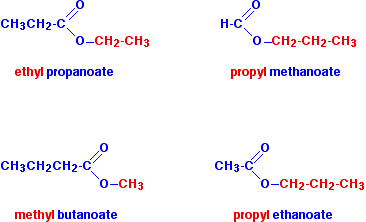Ester Formationsource : chemguide.co.uk

Ester Formation –

The reacn between alc. & acid to form ester is an homogeneous equilibrium in liquid system.

Let ‘a’ moles of Acetic acid &’ b’ moles of ethyl Alcohol ,at equilibrium x moles of ester & x moles of water are formed i.e  x moles of CH3COOH & x moles of alcohol have been consumed. Let ‘V’ be the total volume.

CH3COOH(l) + C2H5OH(l)CH3COOC2H5 (l)+H2O (l)

a/V                   b/V                               0                             0

(a-x)/V            (b-x)/V                         x/V                           x/V

Applying law of mass action,

Kc=[ester][water]/[acid][alcohol]

=[ (x/V) (x/V)]/[c/V)(b-x)/V)]

Kc  = x2/(a-x) (b-x)

1. In an experiment 1 mole of Acetic Acid & 1 mole of alc. were allowed to react until equilibrium was established. The equilibrium mixture was found to contain 2/3 mole of ester. Calculate the equilibrium constt.

Ans.                CH3COOH(l) + C2H5OH(l)CH3COOC2H5 (l)+H2O (l)

Initial Conc               1                      1                      0                              0

At Equi.                     1-2/3               1-2/3               2/3                         2/3

=1/3                =1/3

Kc=[ester][water]/[acid][alcohol]

=[ (2/3) (2/3)]/[(1/3)(1/3)]

Kc  =4 Ans.

Q.2      At constt. temperature 30 gm. acetic acid & 23 gm. of ethyl alcohol react together. At equilibrium 6gm. H2O is formed Cal. Kc=?

Ans.    w of CH3COOH= 30gm

m of CH3COOH=60

w of C2H5OH = 23gm

m of C2H5OH = 46

Initial Concn of CH3COOH = 30/60 mole/l = 1/2 mole/l

Initial Concn of C2H5OH= 23/46 mole/l = 1/2  mole/l

At equilibrium,

Conc. of H2O =6/18  mole/l  = 1/3 mole/l

CH3COOH       +         C2H5OHCH3COOC2H5  + H2O

Initial Conc.       1/2                                1/2                                0                            0

At. Equi.           ( 1/2)-(1/3)                 ( 1/2)-(1/3)                    1/3                      1/3

( mole/l)              =1/6                           =1/6

Kc=[ester][water]/[acid][alcohol]

=[ (1/3) (1/3)]/[(1/6)(1/6)]

Kc  =4 Ans.

Q.3      In the reacn

A+B C+D.      The initial concentration of A & B are 0.8 mole/l. At equilibrium concentration of C is 0.06 mole/l. Calculate Kc.

Ans.                                        A         +          BC         +          D

Initial conc. (mole/l)          0.8                   0.8                   0                      0

at Equi.                                 0.8-0.6            08.-0.6           0.6                   0.6

= 0.2               =0.2

Kc =[C][D]/[A][B]

=  [0.6 x 0.6 ]/[0.2 x 0.2]

=9 Ans.

1. In an experiment at 1000C 1 mole of acetic acid, 1 mole of ethyl A/c & 1 mole of H2O are taken. At equilibrium 54.3 % acid is converted into ester. Calculate the Kc=?

Ans.    Initial moles of acetic acid=1 mole

Initial moles of C­2H5OH=1 mole

Initial moles of H2O = 1mole

At equilibrium,

moles of Acid converted to ester=( 1 x 54.3) /100 =0.543 mole

CH3COOH + C2H5OHCH3COOC2H5  + H2O

Initial Conc.          1                       1                                 0                       1

At. Equi.              (  1-0.543)/V      (  1-0.543)/V     0.543/V              (  1 +0.543)

=1.543/V

Kc=[ester][water]/[acid][alcohol]

=  [ (0.543 )  (1.543)] /  [(0.457 )(0.457)]

Kc    =    4.0 Ans.